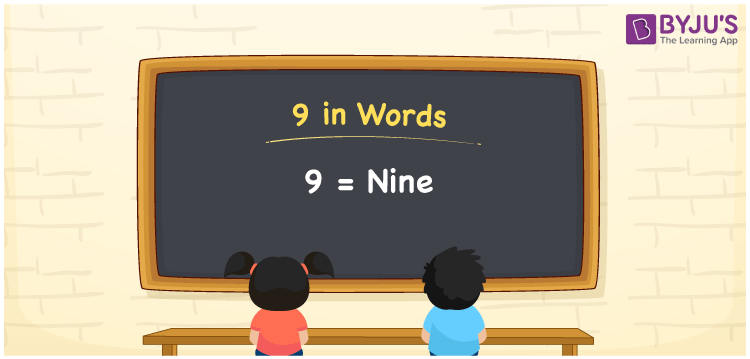# 9 in Words

9 in words is written as Nine. Using the place value chart, we can derive any number in words. Suppose if you have 9 candies, then you can write as “I have Nine candies with me”. 9 is a cardinal number or counting number as it denotes a specific quantity.

 9 in words Nine Nine in numerical form 9

## 9 in English Words

Generally numbers in words are written using English alphabets. Therefore, in English, the number 9 is written as Nine.## How to Write 9 in Words?

Place value charts are necessary to derive any number to words. Let us write a given number using a place value chart.

 Tens Ones 0 9

Therefore, we can write the expanded form as:

0 x Tens + 9 x Ones

= 0 x 10 + 9 x 1

= 0 + 9

= 9

= Nine

Hence, 9 in words is written as Nine.

Interesting way of writing 9 in words

0 = zero

9 = Nine

0 + 9 = 9

9 = Nine

Zero + Nine = Nine

9 is a natural number. The successor of the number 9 is 10 whereas the predecessor of 9 is 8

• 9 in words – Nine
• Is 9 an odd number? – Yes
• Is 9 an even number? – No
• Is 9 a perfect square number? – Yes
• Is 9 a perfect cube number? – No
• Is 9 a prime number? – No
• Is 9 a composite number? – Yes

## Frequently Asked Questions on 9 in Words

Q1

### How to write 9 in English?

In English, 9 is written as Nine.
Q2

### Write the value of 1 + 8 in words.

1 + 8 = 9 One + Eight = Nine Hence, 1 + 8 is equal to Nine.
Q3

### How do you write Rs. 9 in words?

We can write Rs. 9 in words as Nine rupees.